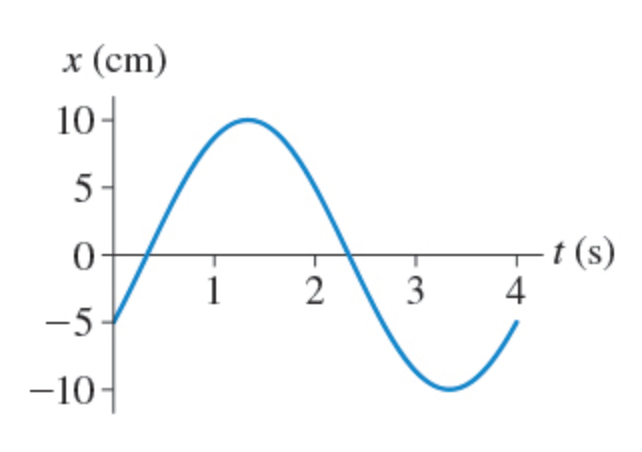# Problem: a) What is the amplitude of the oscillation shown in (Figure 1)?b) What is the frequency of the oscillation shown in the figure?

###### FREE Expert Solution

Frequency:

$\overline{){\mathbf{f}}{\mathbf{=}}\frac{\mathbf{1}}{\mathbf{T}}}$

where T is the periodic time.

(a)

Amplitude is the maximum displacement from the mean position. The mean position is 0cm.

82% (488 ratings)###### Problem Detailsa) What is the amplitude of the oscillation shown in (Figure 1)?

b) What is the frequency of the oscillation shown in the figure?